## Fractal Dimension - Sierpinski GasketDetermining the capacity dimension of the Sierpinski gasket is a good starting point because we can easily retrieve the appropriate values for P and S in our formula by examining the fractal image after a few iterations. This image is shown on the left. Observe that it is a triangle, and consists of three smaller triangles with a triangular space between them. These three triangles are identical copies of the entire fractal, so we decide that our value for P will be 3. Now what is the scaling factor? Observation also easily tells us that the edge of the smaller triangles is half the edge of the fractal itself. Thus our scaling factor S is 2. Applying these to our formula, we get: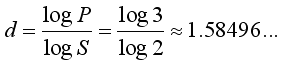We came to this result incredibly easily. Note that we have arrived at an irrational result for the gasket's dimension, which conceptually is hard to fathom. However we should not be surprised that many values will produce a non-integral capacity dimension, since our formula involves the ratio of two logarithms.

The key to the ease with which we came up with our P and S values is that the gasket is infinitely complex. The fractal is made up of three exact copies of itself, and thus each of the three smaller triangles is constituted of three smaller gaskets, and so on. If we simply draw three filled equilateral triangles, and stack them in the same way to produce a larger equilateral triangle as shown below, we cannot conclude that the larger triangle has the same dimension as the Sierpinski gasket, because the smaller triangles are definitely not exact copies of the larger one. This figure is actually the second iteration of the gasket, when certain Lindenmayer grammars are used to describe it. The fractal itself is the limit as the number of iterations approaches infinity.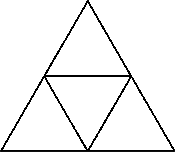Our capacity dimension formula does not apply to this figure because it is not self-similar.

Note that our value for d is between 1 and 2. From this arises one argument for the validity of the formula for capacity dimension. We observe that because the gasket is infinitely complex, the limit of its length is infinite (a short proof is given below). However, because the gasket is just a line after all (the white area is not part of the gasket), it has zero area. An infinite length suggests a dimension greater than 1, but an area of zero suggests a dimension less than 2, and our result agrees with this. Furthermore, our value for d suggests that the Sierpinski gasket is a little "closer" to being 2-dimensional than it is to being 1-dimensional.

### Proof of Infinite Length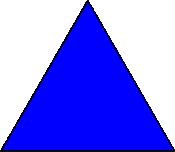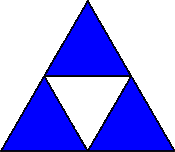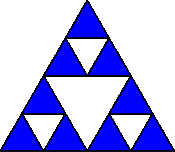n = 0 Smallest triangles: 1 Initial line length is 1. n = 1 Smallest triangles: 3 1 triangle is split into 3 by drawing 3 lines of length 1/2. n = 2 Smallest triangles: 9 3 triangles are split into 9 by drawing 9 lines of length 1/4.

We let n be the number of iterations performed so far, with n = 0 for the first iteration. Observe the first three iterations illustrated above. The first iteration consists of 1 triangle. This is then split into 3 smaller triangles (the centre one doesn't count). Each of these 3 is then split to produce 9 triangles. If we only consider the smallest triangles, at each iteration n there are 3^n of them. These smallest triangles are split into 3 triangles by inscribing three lines inside each: between the midpoints of the edges. These inscribed lines are each 1/2 the length of the triangle being split. If the length of the first triangle's edges is 1, then at n = 1 the inscribed edges have length 1/2 and at n = 2 they have length 1/4, and at iteration n they have length (1/2)^n. So at iteration n, the 3^(n-1) smallest triangles from the previous iteration are split by inscribing 3 edges with length (1/2)^n. Thus at iteration n the length is increased by 3^(n-1)*3*(1/2)^n = (3/2)^n. At n = 0 the length is 3, thus we achieve the formula for the length of the Sierpinski gasket as an infinite sum: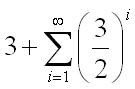Since the terms in the summation increase as i increases, the sum is divergent and thus the length of the Sierpinski gasket is infinite. QED